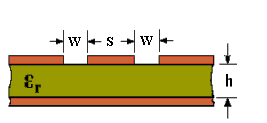# Coplanar Waveguide With Ground Characteristic Impedance Calculator

The characteristic impedance (Zo) of coplanar waveguide with ground or microstrip lines with signal side ground plane can be calculated using the active calculator or the equations at the bottom of the page.INPUT DATA Relative Dielectric Constant ( εr ): Track Width (S): mm Gap Width (W): mm Dielectric Thickness (h): mm
 RESULTS Effective Dielectric Constant (εeff): Characteristic Impedance (Zo): Ohms

This calculator uses JavaScript and will function in most modern browsers. For more information see About our calculators

The original equations are in Transmission Line Design Handbook by Brian C Wadell, Artech House 1991 page 79. The equations use "a" for the track width and "b" for the sum of the track width plus the gaps either side.To avoid microstrip line modes, it is recommended that h >> b and that the component side ground extend away from the track on each side more than "b".

Note: For those who wish to understand how K(k) is found, there is a calculator and equations for Elliptical Integrals of the First Kind

This calculator is provided free by Chemandy Electronics in order to promote the FLEXI-BOX